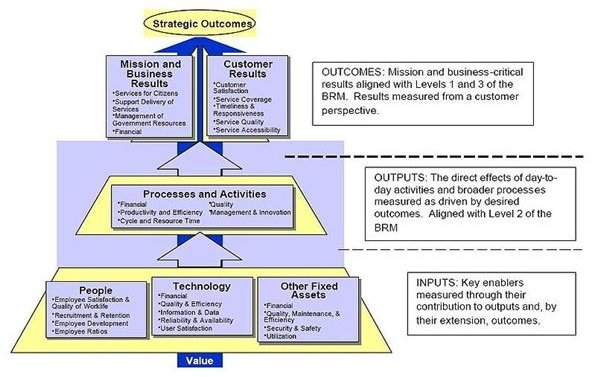# The Significance of Double-Declining Depreciation as a Cost Recovery Tool

## What is Double-Declining Depreciation?Double-declining depreciation is a method of calculating depreciation costs that stem from the declining balance depreciation. Details about the latter were discussed in a separate article Declining-Balance Depreciation: Explanations and Examples. The rationale behind these two methods is the allocation or recovery of acquisition cost based on the performance of the fixed asset during the peak years of its useful life, and not necessarily on the number of years that the asset will be put into use.

In using this method, the same mechanics of using the net book value (acquisition cost less accumulated depreciation cost) as the base amount for calculating the annual depreciation is observed.

However, the rate of annual depreciation will be doubled by multiplying the depreciation rate at 200% instead of 100%.

At this point, the significance of the doubled effect is still unclear; hence explanations and examples for this depreciation method illustrate its calculations and effect.

## Example of the Double-Declining Depreciation Calculation

The following givens are used as the basis for computations:

A complete set of office furniture is bought on January 1 at an acquisition cost of \$50,000 and has an estimated useful life of 8 years based on IRS standards for estimated useful life. A scrap value of \$2,000 will be recognized at the end of the 8th year.

Rate of Annual Depreciation formula for this equipment = 1/8 x 200%

= 0.125 x 200% = 25%

Rate of Annual Depreciation = 25%

Computation of Yearly Depreciation Costs Based on Double-Declining Depreciation Method

Similar to the mechanics of the declining balance depreciation, you have to refer to the running balance of the accumulated depreciation account to determine the amount deducted from the acquisition cost.

• 1st year = \$50,000 x 25% = \$12,500
• 2nd year = (\$50,000 – \$12,500) x 25% = \$37,500 x 25% = \$9,375
• 3rd year = (\$50,000 – \$21,875) x 25% = \$28,125 x 25% = \$7,031
• 4th year = (\$50,000 – \$28,906) = \$21,094 x 25% = \$5,273
• 5th year = (\$50,000 – \$34,179) x 25% = \$15,821 x 25% = \$3,955
• 6th year = (\$50,000 – \$38,134) x 25% = \$11,866 x 25% = \$2,966
• 7th year = (\$50,000 – \$41,100) x 25% = \$8,900 x 25% = \$2,225
• 8th year = (\$50,000 – \$43,325) x 25% = \$6,675 – Scrap Value of \$5,000 = \$1,675

Running Balance of Accumulated Depreciation:

• 1st year = 0.00 + \$12,500 = \$ 12,500
• 2nd year = \$12,500 + \$9,375 = \$21, 875
• 3rd year = \$21,875 + \$7,031 = \$28,906
• 4th year = \$28,906 + \$5,273 = \$34,179
• 5th year = \$34,179 + \$3,955 = \$38,134
• 6th year = \$38,134 + \$2,966 = \$41,100
• 7th year = \$41,100 + \$2,225 = \$43,325
• 8th year = \$43,325 + \$1,675 = \$45, 000

## Explaining the Effect of Double-Declining Depreciation by Comparing It vs. the Straight-Line Method

In order to appreciate the significance of this method of depreciation, let us compute the annual depreciation costs produced by the straight-line method.

Annual Depreciation – Straight Line = (\$50,000 – S.V. \$5,000) / 8 years' estimated life

= \$45,000 / 8 years

= \$5,625 per year

In comparing the total depreciation expense for the first three years, it shows that had the straight-line method been used, accumulated depreciation would have amounted to only \$16,875; as against the amount of \$28,906 accumulated by the double-declining method as of the 3rd year. Hence, cost recovery was faster during the asset’s peak years.

Further compare the total amount of depreciation recognized from the 4th year to the 8th year:

• Straight-Line method = 5 years x \$5,625 = \$28,125
• Double Declining Method = \$45,000 – \$28,906 = \$16,094

The significance of this method is that the allocated costs become much lower at a time when cost of repairs becomes inevitable–hence lessening the burden of cost allocation during these periods.

Based on the US GAAP Accounting Study Guide, the double-declining method can be modified by multiplying the fraction of years by 150% instead of 200%.

Image Credit: Performance Reference Model